# A spring, a rubber band, and chaos

Suppose you have a mass suspended by the combination of a spring and a rubber band. A spring resists being compressed but a rubber band does not. So the rubber band resists motion as the mass moves down but not as it moves up. In  the authors use this situation to motivate the following differential equation:whereIf ab then we have a linear equation, an ordinary damped, driven harmonic oscillator. But the asymmetry of the behavior of the rubber band causes a and b to be unequal, and that’s what makes the solutions interesting.

For some parameters the system exhibits essentially sinusoidal behavior, but for other parameters the behavior can become chaotic.

Here’s an example of complex behavior.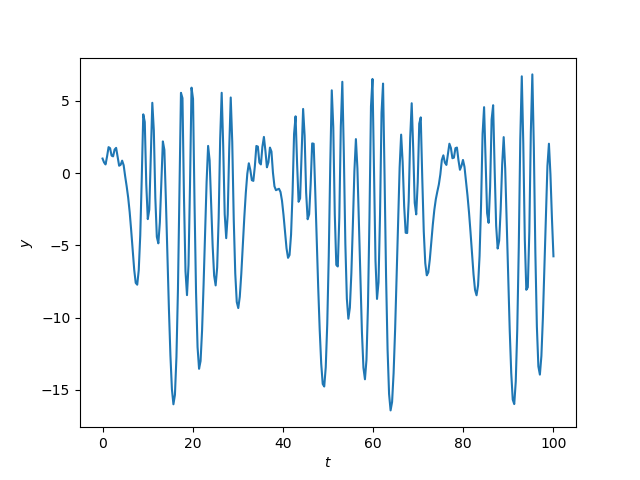Here’s the Python code that produced the plot.

    from scipy import linspace, sin
from scipy.integrate import solve_ivp
import matplotlib.pyplot as plt

def pos(x):
return max(x, 0)

def neg(x):
return max(-x, 0)

a, b, λ, μ = 17, 1, 15.4, 0.75

def system(t, z):
y, yp = z # yp = y'
return [yp, 10 + λ*sin(μ*t) - 0.01*yp - a*pos(y) + b*neg(y)]

t = linspace(0, 100, 300)

sol = solve_ivp(system, [0, 100], [1, 0], t_eval=t)
plt.plot(sol.t, sol.y)
plt.xlabel("$t$")
plt.ylabel("$y$")


In a recent post I said that I never use non-ASCII characters in programming, so in the code above I did. In particular, it was nice to use λ as a variable; you can’t use lambda as a variable name because it’s a reserved keyword in Python.

Update: Here’s a phase portrait for the same system.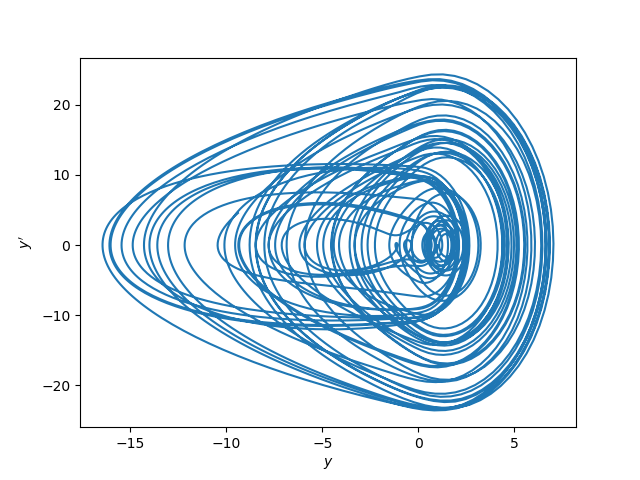L. D. Humphreys and R. Shammas. Finding Unpredictable Behavior in a Simple Ordinary Differential Equation. The College Mathematics Journal, Vol. 31, No. 5 (Nov., 2000), pp. 338-346

# A brief comment on hysteresis

You might hear hysteresis described as a phenomena where the solution to a differential equation depends on its history. But that doesn’t make sense: the solution to a differential equation always depends on its history. The solution at any point in time depends (only) on its immediately preceding state. You can take the state at any particular time, say position and velocity, as the initial condition for the solution going forward.

But sometimes there’s something about the solution to a differential equation that does not depend on its history. For a driven linear oscillator, the frequency of the solution depends only on the frequency of the driving function. But this is not necessarily true of a nonlinear oscillator. The same driving frequency might correspond to two different solution frequencies, depending on whether the frequency of the driving function increased to its final value or decreased to that value.

Hysteresis is interesting, but it’s more remarkable that linear system does not exhibit hysteresis. Why should the solution frequency depend only on the driving frequency and not on the state of the system?

The future of system with hysteresis still depends only on its immediately preceding state. But there may be a thin set of states that lead to solutions with a given frequency, and the easiest way to arrive in such a set may be to sneak up on it slowly.

# A better adaptive Runge-Kutta method

This is the third post in a series on Runge-Kutta methods. The first post in the series introduces Runge-Kutta methods and Butcher tableau. The next post looked at Fehlberg’s adaptive Runge-Kutta method, first published in 1969. This post looks at a similar method from Dormand and Prince in 1980.

Like Fehlberg’s method, the method of Dormand and Prince can be summarized in a big, intimidating tableau, which we will display below. However we will discuss three differences between the methods:

• Order 4(5) vs 5(4)
• Derivative reuse
• Precision / computation ratio

## Dormand Prince tableau

Here’s the Butcher tableau for the Dormand-Prince method in all it’s glory:The only detail of the table that will be important below is that 7th and 8th rows are identical.

## Order 4(5) vs order 5(4)

Fehlberg’s method, a.k.a. RKF45, computes each update to the solution using a 4th order Runge-Kutta method, and uses a 5th order Runge-Kutta method to estimate the error.

The method of Dormand and Prince also uses 4th and 5th order Runge-Kutta methods, but in the opposite way. The fifth order method is used to advance the solution, and the 4th order method is used for comparison to estimate error.

## Derivative reuse

The work in solvingby a Runge-Kutta method is roughly proportional to the number of stages. Dormand-Prince is a 7-stage method while Fehlberg is a 6-stage method, so it would seem that the latter is more efficient. However, if you look back at the Dormand-Prince tableau, the last row above the horizontal line equals the first row below the line. That means that the last evaluation of f at one step can be reused at the first evaluation of f at the next step.

## Precision per unit work

In their book Solving Differential Equations, vol. 1, Hairer et al compare several adaptive Runge-Kutta methods, including Fehlberg (RKF45) and Dormand-Prince, and conclude that the latter produces more precision per unit work.

We again see that the [Fehlberg] method underestimates the local error. Further, with the use of local extrapolation, the advantage of RKF4(5) melts away to a large extent. The best method of all these is without a doubt the coefficient set of Dormand and Prince.

# How to estimate ODE solver error

This post brings together several themes I’ve been writing about lately: caching function evaluations, error estimation, and Runge-Kutta methods.

A few days ago I wrote about how Runge-Kutta methods can all be summarized by a set of numbers called the Butcher tableau. These methods solveby evaluating f at some partial step, then evaluating f again at some new partial step and some linear combination of values from previous steps, etc. In the preface to J. C. Butcher’s book on differential equations, JM Sanz-Serna describes this structure as follows.

Runge-Kutta schemes … are highly nonlinear with a remarkable Matrioshka doll structure, where the vector field has to be evaluated an an expression that involves the vector field evaluated at and expression that involves the vector field …

Once all the “Matrioshka dolls” are lined up, i.e. all the intermediate results have been calculated, the final estimate is a linear combination of these values.

Here’s the clever idea behind adaptive solvers: Create two Runge-Kutta methods of different orders that depend on the same intermediate results. Then both can be computed without new function evaluations, and the results compared. The difference between the results can be used as an estimate of the local error. Then you can adjust your step size accordingly and try again.

I’m going to present two adaptive Runge-Kutta schemes. I’ll go over Fehlberg’s method in this post and a variation in the next post that has some advantages over Fehlberg’s method.

## Runge-Kutta-Felhberg (RKF45)

Fehlberg’s method, commonly known as RKF45, starts with a six-stage Runge Kutta method whose coefficients are given by the following tableau.The meaning of the tableau is described here. (Imagine the effort it took Erwin Felhberg to derive this in 1969. Presumably he had little more than a desktop calculator to help. Maybe not even that.)

What’s important about the tableau for this post is that the coefficients above the horizontal line are used to create six numbers, the k‘s in the notation of the post referenced above. The k‘s are multiplied by the coefficients below the horizontal line to produce the solution of the differential equation at the next step. This is the “4” of RKF45.

RKF45 then applies another method which reuses all the k‘s in a different linear combination. This is summarized in the following variation of the Butcher tableau.This is essentially two tableau combined into one. The first is as above. The second is like the one above but with a different bottom row. The bottom row gives the coefficients for a Runge-Kutta method corresponding to the “5” part of RKF45.

The 4 stands for 4th order, i.e. the local error for a step size h is O(h4). The 5 stands for 5th order, i.e. local error O(h5). RKF45 is two different methods, but they share so much computation that the second one almost comes for free; it does not require any new function evaluations, only taking a linear combination of six numbers.

However, RK45 is only “free” if you’ve gone to the effort of using a six-stage method. The amount of computation is roughly proportional to the number of stages, so we do about 50% more work to have RKF45 with an error estimate than doing the most common 4th order RK method. So if you knew exactly what step size to use, basic RK would be more efficient. But how could you know the optimal step size a priori?

By guiding us to choose the right step size, the extra work in RKF45 more than pays for itself. It could save a lot of computation that would come from using too small a step size, or prevent inaccurate results due to using too large a step size. Or maybe both: maybe a differential equation needs small steps at one period of time and can use larger steps at another period of time.

For more information on Felhberg’s method, see Solving Differential Equations by Hairer et al.

# Runge-Kutta methods and Butcher tableau

If you know one numerical method for solving ordinary differential equations, it’s probably Euler’s method. If you know two methods, the second is probably 4th order Runge-Kutta. It’s standard in classes on differential equations or numerical analysis to present Euler’s method as conceptually simple but inefficient introduction, then to present Runge-Kutta as a complicated but efficient alternative.

Runge-Kutta methods are a huge family of numerical methods with a wide variety of trade-offs: efficiency, accuracy, stability, etc. Euler’s method is a member of the Runge-Kutta family as are countless other variations. You could devote a career to studying Runge-Kutta methods, and some people have.

Beneath the complexity and variety, all Runge-Kutta methods have a common form that can be summarized by a matrix and two vectors. For explicit Runge-Kutta methods (ERK) the matrix is triangular, and for implicit Runge-Kutta methods (IRK) the matrix is full.

This summary of an RK method is known as a Butcher tableau, named after J. C. Butcher who classified RK methods.

## “The” Runge-Kutta method

For example, let’s start with what students often take to be “the” Runge-Kutta method. This method approximates solutions to a differential equation of the formbywhereThe Butcher tableau for this ERK method isThe numbers along the left side are the coefficients of h in the first argument of f.

The numbers along the bottom are the coefficients of the ks in the expression for the value of y at the next step.

The numbers in the middle of the array are the coefficients of the ks in second argument  of f. Because this is an explicit method, each k only depends on the previous ks, and so the table of coefficients has a triangular form.

## Runge-Kutta 3/8 rule

The method above is the most common 4th order ERK rule, there is another known as the 3/8 rule. It is a little less efficient and a little more accurate. A step of this rule is given bywhereThis method is summarized in the following Butcher tableau.This example makes it a little easier to see what’s going on since none of the coefficients in the triangular array are zero. Full detail is given in the section below.

## General Explicit Runge-Kutta

The most general form of an ERK rule with s steps iswhereand the Butcher tableau is## General implicit Runge-Kutta

With explicit (ERK) methods, each k depends only on its predecessors. With implicit (IRK) methods each k potentially depends on each of the others. The matrix in the tableau is full, not triangular, and one must solve for the ks.

Nowwith the sum going all the way up to s, and the Butcher tableau isImplicit methods are more complicated to implement, and require more computation for a given step size. However, they are more stable for stiff differential equations and may allow larger steps. Implicit methods are less efficient when they’re not needed, and more efficient when they are needed.

## Back to Euler’s method

I said at the top of the post that Euler’s method was a special case of Runge-Kutta. The Butcher tableau for the explicit (forward) Euler method is simplyand the tableau for the implicit (backward) Euler method is justIn this post I say more about these two methods and compare their stability.

# The orbit that put men on the moon

Richard Arenstorf (1929–2014) discovered a stable periodic orbit between the Earth and the Moon which was used as the basis for the Apollo missions.

His orbit is a special case of the three body problem where two bodies are orbiting in a plane, i.e. the Earth and the Moon, along with a third body of negligible mass relative to the other bodies, i.e. the satellite.

The system of differential equations for the Arenstorf orbit arewhereHere the Earth is at the origin and the Moon is initially at (0, 1). The mass of the Moon is μ = 0.012277471 and the mass of the Earth is μ’ = 1-μ.

The initial conditions areHere’s a plot of the orbit.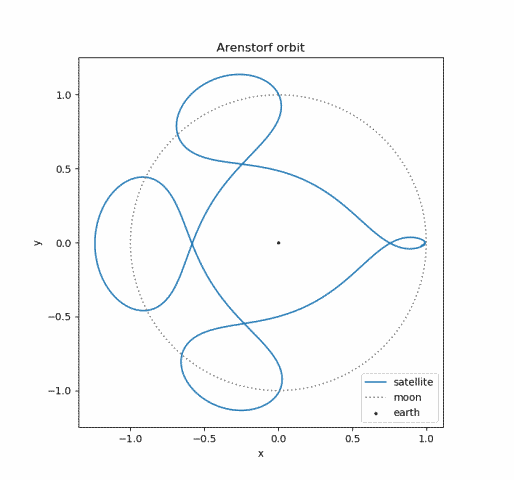I found the equations above in  which sites the original paper . Note that the paper was written in 1963, seven years before the Apollo missions. Also, before leaving NASA Arenstorf mapped out a rescue orbit. This orbit was later used on Apollo 13.

## Richard Arenstorf

I was fortunate to do my postdoc at Vanderbilt before Arenstorf retired and was able to sit in on an introductory course he taught on orbital mechanics. His presentation was leisurely and remarkably clear.

His course was old-school “hard analysis,” much more concrete than the abstract “soft analysis” I had studied in graduate school. He struck me as a 19th century mathematician transported to the 20th century.

He scoffed at merely measurable functions. “Have you ever seen a function that wasn’t analytic?” This would have been heresy at my alma mater.

When I asked him about “Arenstorf’s theorem” from a recently published book I was reading, he said that he didn’t recognize it. I forget now how it was stated, maybe involving Banach spaces and/or manifolds. Arenstorf was much more concrete. He wanted to help put a man on the Moon, not see how abstractly he could state his results.

## More orbital mechanics posts

 Hairer, Nørsett, and Wanner. Solving Ordinary Differential Equations I: Nonstiff Problems. Springer-Verlag 1987.

 Richard F. Arenstorf. Periodic Solutions of the Restricted Three Body Problem Representing Analytic Continuations of Keplerian Elliptic Motion. American Journal of Mathematics, Vol. 85, No. 1 (Jan., 1963), pp. 27–35.

# Stiff differential equations

There is no precise definition of what it means for a differential equation to be stiff, but essentially it means that implicit methods will work much better than explicit methods. The first use of the term  defined stiff equations as

equations where certain implicit methods, in particular BDF, perform better, usually tremendously better, than explicit ones.

We’ll explain what it means for a method to be explicit or implicit and what BDF means. And we’ll give an example where the simplest implicit method performs much better than the simplest explicit method. Here’s a plot for a little foreshadowing.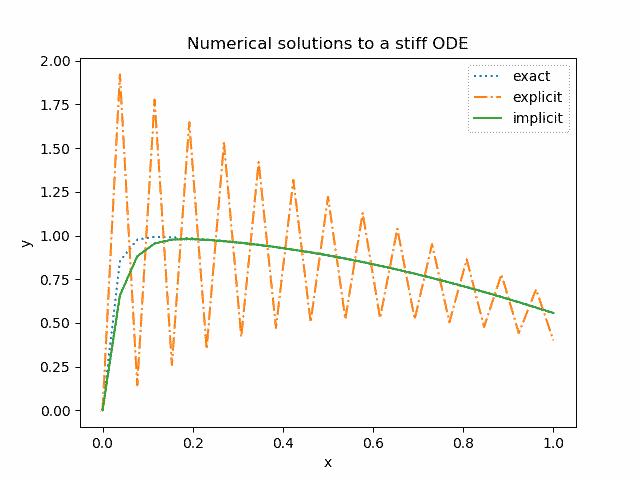## Euler’s method

Suppose you have a first order differential equationwith initial condition y(0) = c. The simplest numerical method for solving differential equations is Euler’s method. The idea is replace the derivative y‘ with a finite differenceand solve for y(x + h). You start out knowing y(0), then you solve for y(h), then start over and use y(h) to solve for y(2h), etc.

## Explicit Euler method

There are two versions of Euler’s method. The explicit Euler method uses a forward difference to approximate the derivative and the implicit Euler method uses a backward difference.

Forward difference means that at a given point x, we approximate the derivative by moving ahead a step hand evaluating the right hand side of the differential equation at the current values of x and y, i.e.and so## Implicit Euler method

The idea of the implicit Euler method is to approximate the derivative with a backward differenceor equivalentlyThe text quoted at the top of the post referred to BDF. That stands for backward difference formula and we have an example of that here. More generally the solution at a given point could depend on the solutions more than one time step back .

This is called an implicit method because the solution at the next time step,appears on both sides of the equation. That is, we’re not given the solution value explicitly but rather we have to solve for it. This could be complicated depending on the nature of the function f. In the example below it will be simple.

## Example with Python

We’ll take as our example the differential equationwith initial condition y(0) = 0.

The exact solution, written in Python, is

    def soln(x):
return (50/2501)*(sin(x) + 50*cos(x)) - (2500/2501)*exp(-50*x)


Here’s the plot again from the beginning of the post. Note that except at the very beginning, the difference between the implicit method approximation and exact solution is too small to see.In the plot above, we divided the interval [0, 1] into 27 steps.

    x = linspace(0, 1, 27)

and implemented the explicit and implicit Euler methods as follows.

    def euler_explicit(x):
y = zeros_like(x)
h = x - x
for i in range(1, len(x)):
y[i] = y[i-1] -50*h*(y[i-1] - cos(x[i]))
return y

def euler_implicit(x):
y = zeros_like(x)
h = x - x
for i in range(1, len(x)):
y[i] = (y[i-1] + 50*h*cos(x[i])) / (50*h + 1)
return y


Here’s a plot of the absolute error in both solution methods. The error for the implicit method, too small to see in the plot, is on the order of 0.001.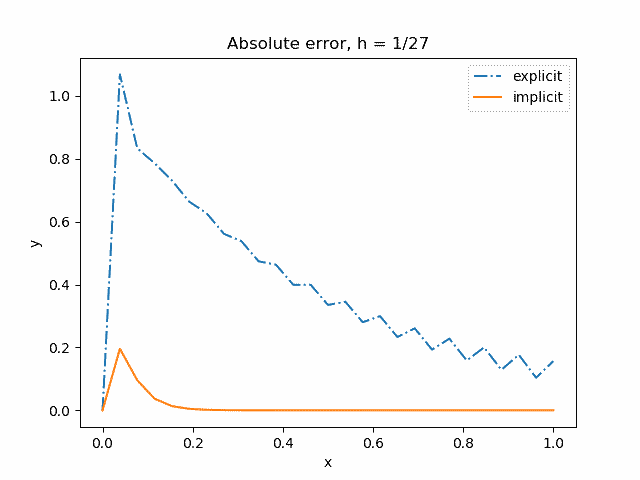In the plots above, we use a step size h = 1/27. With a step size h = 1/26 the oscillations in the explicit method solution do not decay.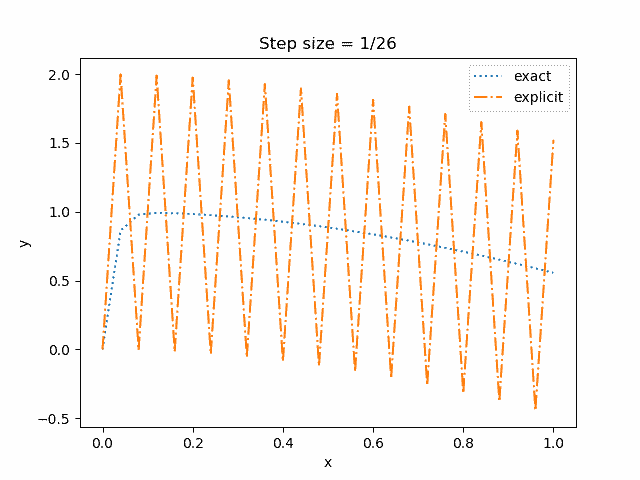And with a step size h = 1/25 the oscillations grow.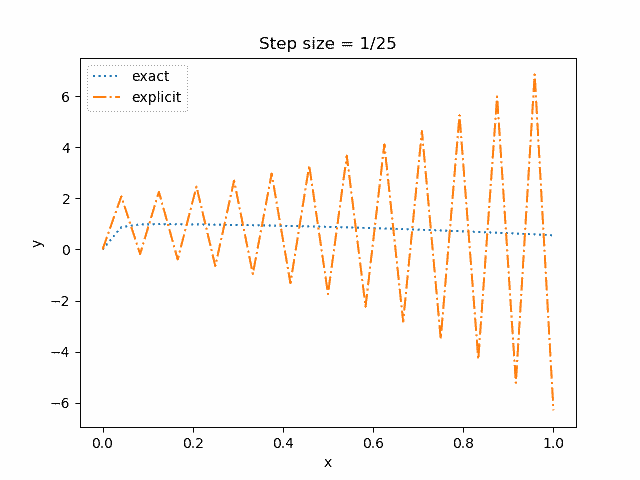The explicit method can work well enough if we take the step size smaller, say h = 1/50. But smaller step sizes mean more work, and in some context it is not practical to use a step size so small that an explicit method will work adequately on a stiff ODE. And if we do take h = 1/50 for both methods, the error is about 10x larger for the explicit method.

By contrast, the implicit method does fairly well even with h as large as 1/5.

(The “exact” solution here means the analytical solution sampled at six points just as the numerical solution is available at six points. The actual solution is smooth; the sharp corner comes from sampling the function at too few points for it to look smooth.)## Related posts

 C. F. Curtiss and J. O. Hirschfelder (1952). Integration of stiff equations. Proceedings of the National Academy of Sciences. Vol 38, pp. 235–243.

 This may be confusing because we’re still evaluating y at x + h, and so you might think there’s nothing “backward” about it. The key is that we are approximating the derivative of y at points that are backward relative to where we are evaluating f(x, y). On the right side, we are evaluating y at an earlier point than we are evaluating f(x, y).

# A different view of the Lorenz system

The Lorenz system is a canonical example of chaos. Small changes in initial conditions eventually lead to huge changes in the solutions.

And yet discussions of the Lorenz system don’t simply show this. Instead, they show trajectories of the system, which make beautiful images, but do not demonstrate the effect of small changes to initial conditions. Or they demonstrate it in two or three dimensions where it’s harder to see.

If you’ve seen the Lorenz system before, this is probably the image that comes to mind.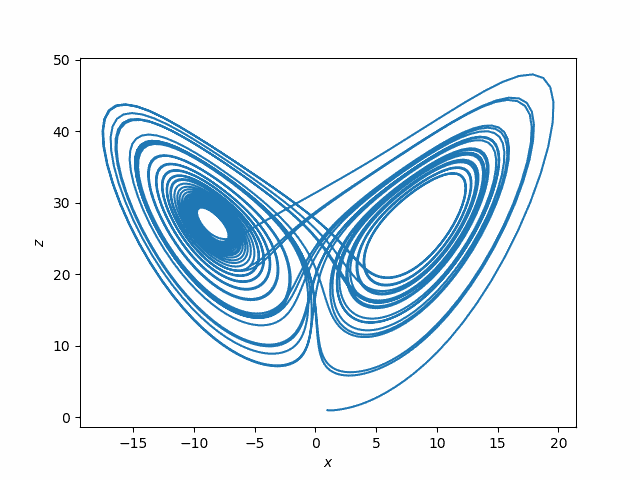This plots (x(t), z(t)) for the solutions to the system of differential equations

x‘= σ(yx)
y‘ = x(ρ – z) – y
z‘ = xy – βz

where σ = 10, ρ = 28, β = 8/3. You could use other values of these parameters, but these were the values originally used by Edward Lorenz in 1963.

The following plots, while not nearly as attractive, are more informative regarding sensitive dependence on initial conditions.In this plot, x1 is the x-component of the solution to the Lorenz system with initial condition

(1, 1, 1)

and x2 the x-component corresponding to initial condition

(1, 1, 1.00001).

The top plot is x1 and the bottom plot is x1x2.

Notice first how erratic the x component is. That might not be apparent from looking at plots such as the plot of (x, z) above.

Next, notice that for two solutions that start off slightly different in the z component, the solutions are nearly identical at first: the difference between the two solutions is zero as far as the eye can tell. But soon the difference between the two solutions has about the same magnitude as the solutions themselves.

Below is the Python code used to make the two plots.

    from scipy import linspace
from scipy.integrate import solve_ivp
import matplotlib.pyplot as plt

def lorenz(t, xyz):
x, y, z = xyz
s, r, b = 10, 28, 8/3. # parameters Lorentz used
return [s*(y-x), x*(r-z) - y, x*y - b*z]

a, b = 0, 40
t = linspace(a, b, 4000)

sol1 = solve_ivp(lorenz, [a, b], [1,1,1], t_eval=t)
sol2 = solve_ivp(lorenz, [a, b], [1,1,1.00001], t_eval=t)

plt.plot(sol1.y, sol1.y)
plt.xlabel("$x$")
plt.ylabel("$z$")
plt.savefig("lorenz_xz.png")
plt.close()

plt.subplot(211)
plt.plot(sol1.t, sol1.y)
plt.xlabel("$t$")
plt.ylabel("$x_1(t)$")
plt.subplot(212)
plt.plot(sol1.t, sol1.y - sol2.y)
plt.ylabel("$x_1(t) - x_2(t)$")
plt.xlabel("$t$")
plt.savefig("lorenz_x.png")


One important thing to note about the Lorenz system is that it was not contrived to show chaos. Meteorologist and mathematician Edward Lorenz was lead to the system of differential equations that bears his name in the course of his work modeling weather. Lorenz understandably assumed that small changes in initial conditions would lead to small changes in the solutions until numerical solutions convinced him otherwise. Chaos was a shocking discovery, not his goal.

# ODE bifurcation example

A few days ago I wrote about bifurcation for a discrete system. This post looks at a continuous example taken from the book Exploring ODEs.

We’ll consider solutions to the differential equationfor two different initial conditions: y(0) = 0.02, y‘(0) = 0 and y(0) = 0.05, y‘(0) = 0.

We’ll solve the ODE with both initial conditions for 0 ≤ t ≤ 600 with the following Python code.

    from scipy import linspace
from scipy.integrate import solve_ivp
import matplotlib.pyplot as plt

def ode(t, z):
y, yp = z
return [yp, 2*(-2 + t/150)*y - 4*y**3]

a, b = 0, 600
t = linspace(a, b, 900)

sol1 = solve_ivp(ode, [a, b], [0.02, 0], t_eval=t)
sol2 = solve_ivp(ode, [a, b], [0.05, 0], t_eval=t)


First let’s look at the solutions for 0 ≤ t ≤ 200.Here’s the code that produced the plots.

    plt.subplot(211)
plt.plot(sol1.t[:300], sol1.y[:300])
plt.subplot(212)
plt.plot(sol2.t[:300], sol2.y[:300])
plt.show()


Note that the vertical scales of the two plots are different in order to show both solutions relative to their initial value for y. The latter solution starts out 2.5x larger, and essentially stays 2.5x larger. The two solutions are qualitatively very similar.

Something unexpected happens if we continue the solutions for larger values of t.Here’s the code that produced the plots.

    plt.subplot(211)
plt.plot(sol1.t, sol1.y)
plt.ylim([-1,1])
plt.subplot(212)
plt.plot(sol2.t, sol2.y)
plt.ylim([-1,1])
plt.show()


Now the vertical scales of the two plots are the same. The solutions hit a bifurcation point around t = 300.

# Driven Van der Pol oscillators

I’ve playing around with driven Van der Pol oscillators.The cosine term is the driving function. You can see a variety of behavior depending on the interaction between the driving frequency and the natural frequency, along with the other parameters. Sometimes you get periodic solutions and sometimes you get chaotic behavior.

Here’s the Python code I started with

    a, b = 0, 300
t = linspace(a, b, 1000)
mu = 9
T = 10

def vdp_driven(t, z):
x, y = z
return [y, mu*(1 - x**2)*y - x + cos(2*pi*t/T)]

sol = solve_ivp(vdp_driven, [a, b], [1, 0], t_eval=t)
plt.plot(sol.y, sol.y)


The first phase plot looked like this:That’s weird. So I increased the number of solution points by changing the last argument to linspace from 1,000 to 10,000. Here’s the revised plot.That’s a lot different. What’s going on? The solutions to the driven Van der Pol equation, and especially their derivative, change abruptly, so abruptly that if there’s not an integration point in the transition zone you get the long straight lines you see above. With more resolution these lines go away. This is understandable when you look at the plot of the solution:and of its derivative:The solution looks fairly regular but the varying amplitudes of the derivative highlight the irregular behavior. The correct phase plot is not as crazy as the low-resolution version suggests, but we can see that the solution does not quickly settle into a limit cycle as it does in the undriven case.